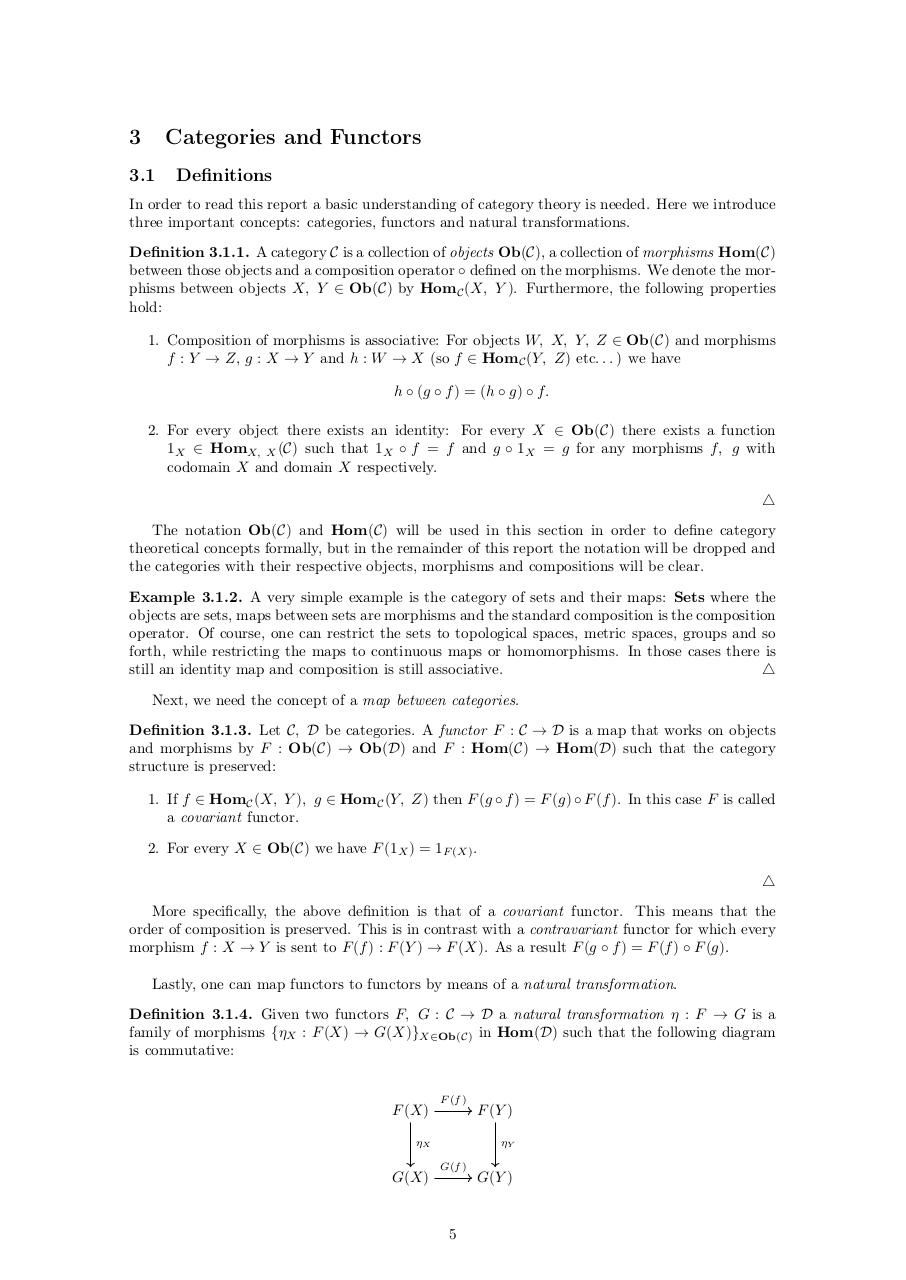# Functors in Differential Geometry.pdfPage 1...3 4 56714

#### Text preview

3

Categories and Functors

3.1

Definitions

In order to read this report a basic understanding of category theory is needed. Here we introduce
three important concepts: categories, functors and natural transformations.
Definition 3.1.1. A category C is a collection of objects Ob(C), a collection of morphisms Hom(C)
between those objects and a composition operator ◦ defined on the morphisms. We denote the morphisms between objects X, Y ∈ Ob(C) by HomC (X, Y ). Furthermore, the following properties
hold:
1. Composition of morphisms is associative: For objects W, X, Y, Z ∈ Ob(C) and morphisms
f : Y → Z, g : X → Y and h : W → X (so f ∈ HomC (Y, Z) etc. . . ) we have
h ◦ (g ◦ f ) = (h ◦ g) ◦ f.
2. For every object there exists an identity: For every X ∈ Ob(C) there exists a function
1X ∈ HomX, X (C) such that 1X ◦ f = f and g ◦ 1X = g for any morphisms f, g with
codomain X and domain X respectively.
4
The notation Ob(C) and Hom(C) will be used in this section in order to define category
theoretical concepts formally, but in the remainder of this report the notation will be dropped and
the categories with their respective objects, morphisms and compositions will be clear.
Example 3.1.2. A very simple example is the category of sets and their maps: Sets where the
objects are sets, maps between sets are morphisms and the standard composition is the composition
operator. Of course, one can restrict the sets to topological spaces, metric spaces, groups and so
forth, while restricting the maps to continuous maps or homomorphisms. In those cases there is
still an identity map and composition is still associative.
4
Next, we need the concept of a map between categories.
Definition 3.1.3. Let C, D be categories. A functor F : C → D is a map that works on objects
and morphisms by F : Ob(C) → Ob(D) and F : Hom(C) → Hom(D) such that the category
structure is preserved:
1. If f ∈ HomC (X, Y ), g ∈ HomC (Y, Z) then F (g ◦ f ) = F (g) ◦ F (f ). In this case F is called
a covariant functor.
2. For every X ∈ Ob(C) we have F (1X ) = 1F (X) .
4
More specifically, the above definition is that of a covariant functor. This means that the
order of composition is preserved. This is in contrast with a contravariant functor for which every
morphism f : X → Y is sent to F (f ) : F (Y ) → F (X). As a result F (g ◦ f ) = F (f ) ◦ F (g).
Lastly, one can map functors to functors by means of a natural transformation.
Definition 3.1.4. Given two functors F, G : C → D a natural transformation η : F → G is a
family of morphisms {ηX : F (X) → G(X)}X∈Ob(C) in Hom(D) such that the following diagram
is commutative:

F (X)

F (f )

ηX

G(X)

F (Y )
ηY

G(f )

5

G(Y )